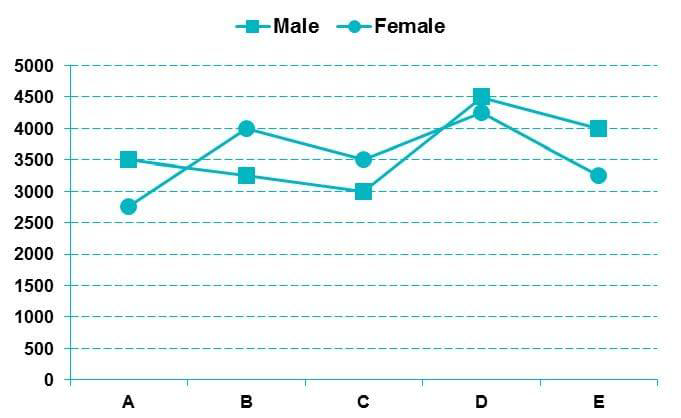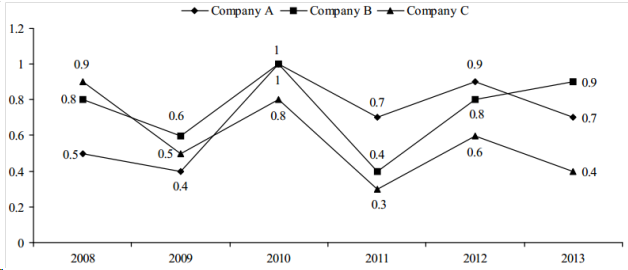# Line Graph

Line Graph - Topic Wise Multiple Choice Questions (MCQ)

Online Topic Wise DI Line Graph MCQ for competitive exam Practice Multiple Choice Questions for IBPS SSC Online Test Private Job Interview

1 )

Directions: Study the following line graph carefully and give answers to questions that follow.

Total number of males and females in five different companiesQue: The number of females from company A is approximately what percent of the total number of females in all the companies put together?

12
13
15
17
19

View Solution

2 )

Directions : Study the following graph carefully to answer these question.

Following line graph shows the ratio of expenditure to income of three companies A, B and C during the period 2008–2013.Per cent profit earned by two companies producing electronic goods over the years

Que:   If the profit earned in 2006 by Company B was Rs. 8,12,500, what was the total income of the company in that year?

Rs. 12,50,000
Rs. 20,62,500
Rs. 16,50,000
Rs. 18,25,000
Rs. 18,45,000

View Solution

3 )

Directions : Study the following graph carefully to answer these question.

Following line graph shows the ratio of expenditure to income of three companies A, B and C during the period 2008–2013.Per cent profit earned by two companies producing electronic goods over the years

Que: If the amount invested by the two companies in 2005 was equal, what was the ratio of the total income of the company A to that of B in 2005?

14:11
34:31
33:31
31:33
21:37

View Solution

4 )

Directions : Study the following graph carefully to answer these question.

Following line graph shows the ratio of expenditure to income of three companies A, B and C during the period 2008–2013.Per cent profit earned by two companies producing electronic goods over the years

Que:   If the amount of profit earned by Company A in 2006 was Rs. 10.15 Lakhs, what was the total investment?

Rs 1605454
Rs 1845000
Rs 1254154
Rs 1845454
Rs 1200054

View Solution

5 )

Directions : Study the following graph carefully to answer these question.

Following line graph shows the ratio of expenditure to income of three companies A, B and C during the period 2008–2013.Per cent profit earned by two companies producing electronic goods over the years

Que: If the amount invested by Company B in 2004 is Rs. 12 Lakhs and the income of 2004 is equal to the investment in 2005, what is the amount of profit earned in 2005 by Company B?

10.23 Lakhs
12.23 Lakhs
11.87 Lakhs
15.23 Lakhs
14.00 Lakhs

View Solution